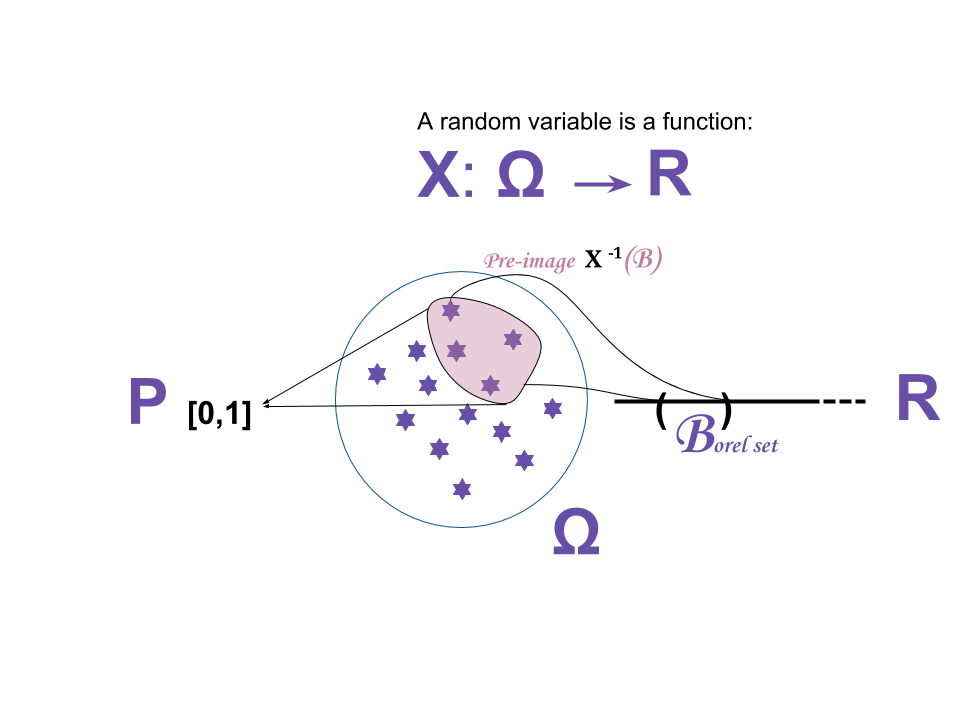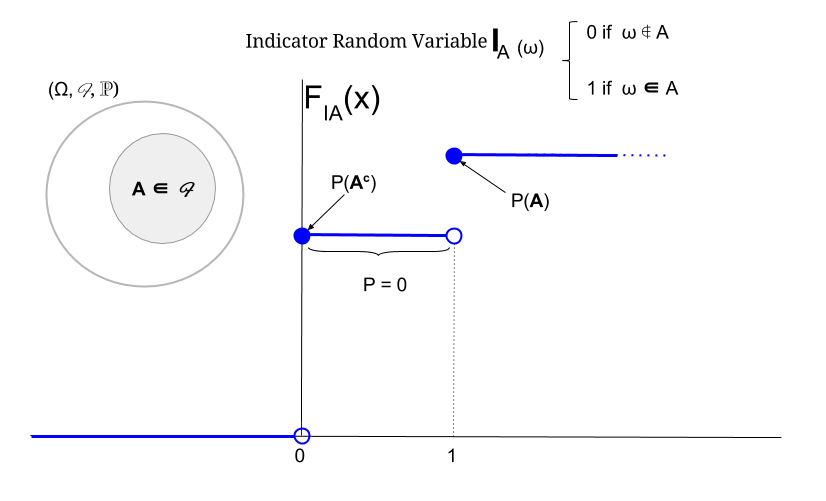### MEASURE THEORY:

#### PROBABILITY SPACES $$(\Omega,\mathcal F, \mathbb P)$$:

1. There are undefined entities: the random experiment (tossing coin) and the outcome.

2. Sample space: Set of all possible outcomes of a random experiment: it can be “heads v tails”, “number of flips in the air”, etc. So the sample space depends on what we are examining.

3. Events: These are the subsets of $$\Omega$$ which are of “interest”. For example, “at least two heads in 3 coin tosses”: $$\bf A=\{HHH\},\{THH\},\{HTH\},\{HHT\}$$. If $$\omega \in \bf A$$ the event has occurred.

An $$\color{blue}{\text{ALGEBRA}}$$ (without the $$\sigma$$) is a collection $$\mathcal F_o$$ of subsets of $$\Omega$$ that fulfills:

1. The null set $$\varnothing \in \mathcal F_o$$;

2. If $$\bf A \in \mathcal F_o$$, then $$\bf A^c \in \mathcal F_o$$ (closed under complementation), which implies $$\varnothing^c = \Omega \in \mathcal F_o$$ and

3. if $$\bf A \in \mathcal F_o$$ and $$\bf B \in \mathcal F_o$$, $$\bf A \cup \bf B \in \mathcal F_o$$ ($$\color{red}{\text{closed under finite unions}}$$), or what is the same, finite intersections).

However, we need more structure than an algebra - “finite unions” is too restrictive. We need a sigma algebra, $$\mathcal F$$, so as to be able to build up all interesting events based on complementary sets and unions. A $$\color{blue}{\text{SIGMA ALGEBRA}}$$:

1. Contains the null set: $$\varnothing \in \mathcal F$$.

2. If $$\bf A \in \mathcal F$$, then $$\bf A^c \in \mathcal F$$ (closed under complementation).

3. If $$\bf A_1, \bf A_2,\cdots$$ is a countable collection of subsets in omega, $$\bigcup\limits_{i=1}^{\infty}A_i \in \mathcal F$$. This symbol means the set of all outcomes ($$\omega$$’s) in at least one of the events ($$A_i$$’s). Of note, $$\bigcup\limits_{i=1}^{\infty}A_i$$ is the same symbol $$\bigcup\limits_{i \in \mathbb N}A_i$$. And this property is expressed as “$$\color{red}{\text{closed under COUNTABLE INFINITE unions}}.$$

Subsets in $$\mathcal F$$ are called $$\mathcal F$$-measurable sets.

The most trivial sigma algebra is $$\mathcal F= \{\varnothing, \Omega\}$$. Other examples are $$\mathcal F = 2^\Omega$$ or $$\mathcal \{\varnothing,\bf A,\bf A^c, \Omega \}$$.

$$(\Omega,\mathcal F)$$ is a measurable space.

What is a MEASURE?

It is a function or map $$\mu: \mathcal F \rightarrow [0,\infty]$$ such that:

1. $$\mu(\varnothing)=0$$.

2. if $$\bf A_1, A_2, \cdots$$ are disjoint $$\mathcal F$$-measurable sets, then: $$\mu\Big(\bigcup\limits_{i=1}^\infty A_i\Big)= \displaystyle \displaystyle \sum_{i=1}^\infty \mu(A_i)$$ (countable additivity).

The triple $$(\Omega,\mathcal F, \mu)$$ is a measure space. If $$\mu(\omega)=1$$, $$\mu$$ is a probability measure. $$\mu = \mathbb P$$, and we’ll talk about probability spaces.

The PROBABILITY MEASURE, $$\mathbb P$$ on $$(\Omega, \mathcal F)$$ is a function $$\mathbb P: \mathcal F \rightarrow [0,1]$$, fulfilling:

1. $$\mathbb P(\varnothing=0)$$;

2. $$\mathbb P(\Omega)=1$$ (this being the only difference to a general measure); and

3. If $$\bf A_1, A_2, \cdots$$ are disjoint $$\mathcal F$$-measurable, $$\mathbb P(A_1 \cup A_2 \cup \cdots) = \mathbb P \Big(\bigcup\limits_{i=1}^\infty A_i \Big)= \displaystyle\sum_{i=1}^\infty \mathbb P(A_i)$$. This is again the principle of countable (finitely or infinitely countable) additivity. Of note, an infinite sum can be expressed as a limit of finite sum: $$\displaystyle\lim_{n\to\infty} \displaystyle \sum_{i=1}^n \mathbb P(A_i)$$.

###### (SOME) PROPERTIES OF PROBABILITY MEASURES:
1. In the last section we see already the continuity of probability measurement property of a probability measure, also expressed as:

If $$A_1,A_2, A_3,\cdots \in \mathcal F$$, which means that they are measurable subsets of the sigma algebra, $$\color{red}{\mathbb P \Big(\bigcup\limits_{i=1}^\infty A_i \Big)=\displaystyle\lim_{n\to\infty} \Big(\displaystyle\sum_{i=1}^n \mathbb P(A_i)\Big)=\displaystyle\lim_{n\to\infty} \mathbb P\Big(\bigcup\limits_{i=1}^n A_i\Big)}$$. Of note, the last step works because of finite additivity (the limit doesn’t count, because it is outside the actual change in notation). The proof is not immediate:

Let $$B_1=A_1$$ and $$B_2=A_2\setminus A_1$$, $$B_3=A_3\setminus(A_2\cup A_1)\cdots$$ until $$B_n=A_n\setminus \bigcup\limits_{i=1}^{n-1}A_i$$. So these are collections of countable sets. 1. $$B_i$$’s are disjoint. And 2. $$\bigcup\limits_{i=1}^\infty A_i= \bigcup\limits_{i=1}^\infty B_i$$. Given these two points we can use countable additivity on $$B_i$$ to get the probability of the unions of $$A_i$$’s:

$$\mathbb P \Big(\bigcup\limits_{i=1}^\infty A_i\Big)= \mathbb P \Big( \bigcup\limits_{i=1}^\infty B_i\Big)=\displaystyle \sum_{i=1}^\infty \mathbb P (B_i)=\displaystyle\lim_{n\to\infty}\Big(\sum_{i=1}^n \mathbb P(B_i)\Big)\underset{\text{finite addi'ty}}{=}\displaystyle\lim_{n\to\infty} \mathbb P\Big(\bigcup\limits_{i=1}^n B_i\Big)=\displaystyle\lim_{n\to\infty} \mathbb P\Big(\bigcup\limits_{i=1}^n A_i\Big) \,\,\square$$

1. Union Bound: If $$A_1, A_2,\cdots$$ are events,$$\mathbb P \Big(\bigcup\limits_{i=1}^\infty A_i\Big) \leq \displaystyle\sum_{i=1}^\infty\mathbb P (A_i)$$. If the equality holds the intersections will have zero probability measure.

#### DISCRETE PROBABILITY SPACES:

The sample space is countable (finite or infinite), and it will either be $$\Omega=\{\omega_1,\omega_2,\cdots,\omega_n\}$$ or $$\Omega=\{\omega_1,\omega_2,\cdots\}$$.

We can afford to take the set of all possible subsets of the sample space as the sigma algebra, $$\mathcal F = 2^\Omega$$, i.e. all subsets of $$\Omega$$ are events, and hence, will have assigned probabilities.

We assign probabilities to all the subsets of $$\Omega$$, or in this case, events $$\bf A$$, via the assigned probabilities to singletons, $$P(\{\omega\})$$.

So, $$P(\bf A)= \displaystyle \sum_{\omega\in A} P(\{\omega\})$$.

Whatever way probabilities are assigned, it should satisfy that $$\displaystyle \sum_{\omega\in \Omega} P(\{\omega\})=1$$.

In the case of a countable infinite sample space, such as the natural numbers $$\mathbb N$$, the powerset has higher cardinality, but it doesn’t matter. The sigma algebra will still be $$\mathcal F= 2^\mathbb N$$, and the singleton will have assigned probaility, for example, $$P(\{k\}) = \frac{1}{2^k}$$, which sum for $$k=1,2,3,\cdots$$ is $$1$$. This is called the geometric measure. In the case of $$\Omega=\{0\}\cup \mathbb N$$ we could assign a Poisson measure.

#### UNCOUNTABLE SAMPLE SPACES:

It is not possible to assign probabilities to $$\mathcal F = 2^\Omega$$ when $$\Omega$$ is uncountable. $$2^\Omega$$ has higher cardinality than the real numbers. We can’t assigned probabilities on, for example, $$[0,1]$$,based on adding up the probabilities of singletons. The most common way to assign probabilities is using intervals $$(a,b)$$ is $$b-a$$. We want $$\mu((a,b)) = \mu((a,b])=\mu([a,b))=\mu([a,b])=b-a$$. We also want the property of translation invariance: $$\bf A \subseteq [0,1]$$, $$\mu(A)=\mu(A\bigoplus x) = \{a+x|a \in A, a+x \leq 1\} \cup \{a + x-1|a \in A, a+x>1\}$$.

We include all intervals (closed and open) in the sigma algebra with their complements, unions and intersections to form a special sigma algebra called a Borel sigma algebra. The intervals are Borel sets.

$$\color{red}{\text{A BOREL SIGMA ALGEBRA is generated by the "interesting subsets", }\mathcal C \text{ (all the open subsets in the real line)}}$$ (or to put aside for now $$\infty$$, the $$[0,1]$$ interval).

There is a theorem stating that there is a unique sigma algebra, which is the smallest sigma algebra generated by $$\mathcal C$$, containing all elements of $$\mathcal C$$. These elements will be all the open intervals in $$[0,1]$$. For any sigma algebra $$\mathcal H$$ that contains $$\mathcal C$$, $$\sigma(\mathcal C) \subseteq \mathcal H=\cap H_i$$ - this $$\sigma(\mathcal C)$$ is the $$\sigma$$-algebra generated by $$\mathcal C$$.

So we take open subintervals of $$\Omega=(0,1]$$ to generate this Borel sigma algebra on $$(0,1]$$ or $$\mathcal B((0,1])$$. This borel sigma algebra has the same cardinality as the real numbers, and contains the sets of practical interest. There are strange sets, such as Vitali’s or Banach-Tarski, which are not included. It will also include the singletons:

$\{b\}= \displaystyle \cap_{n=1}^\infty \big [(b-1/n,\,\, b+1/n) \cap \Omega \big]$.

The closed sets are included also:

$(a,b]=\displaystyle \cap_{n=1}^\infty[(a,\,\,b+1/n)\cap \Omega]$

And the “openess” on the left makes it clear that it is a sigma algebra.

In here Borel sigma algebras are defined as the natural link between $$\sigma$$-algebras for a set $$\bf M$$ and topology: Let $$(\bf M, \mathcal O)$$ be a topological space. Now can we use the choice for open sets in the standard topology to define measurable sets? Yes, and then the generating sets are all the open sets, and the generated sigma algebra, $$\sigma(\mathcal O)$$ is the Borel $$\sigma$$-algebra on $$(\bf M, \mathcal O)$$.

So far we have a Borel $$\sigma$$-algebra on the power set, $$(\Omega,\mathcal B)$$, which has made the construct measurable by reducing the cardinality. But how to give it a measure? The length of any of the open intervals $$(a,b)$$, measured as $$b -a$$ would do, EXCEPT for the fact that we are also including in this Borel sigma algebra some bizarre sets, such as the Cantor set. How to deal with that scattering of points?

We need to extend the measure of these intervals (the Lebesgue measure) to weird sets.

We start with not $$\mathcal F$$, but a collection of subsets of $$\Omega$$ which are finite unions of disjoint intervals of the form $$(a,b]$$ plus $$\varnothing$$. This will be called $$\mathcal F_o$$, and will form an algebra, albeit not a $$\sigma$$-algebra:

$\cup_{n=1}^\infty \big(0,\frac{n}{n+1}\big]=(0,1) \notin \mathcal F_o$.

We have $$\mathcal F_o=\{(a_1,b_2] \cup (a_2,b_2],\cdots\, \cup (a_n, b_n]\}$$, which can be measured as the distances, and then, by Caratheodory’s extension theorem, we can extend this sigma algebra generated by these disjoint intervals ($$\sigma(\mathcal F_o)$$) to prove that it is the same as the Borel sigma algebra on $$(0,1]$$: $$\mathcal B((0,1]))$$.

Caratheodary’s theorem says that if $$\mathcal F_o$$ is an algebra on $$\Omega$$ and $$\mathcal F= \sigma(\mathcal F_o)$$, and $$\mathbb P_o: \mathcal F_o \rightarrow [0,1]$$ such that $$\mathbb P(\Omega = 1)$$ and $$\mathbb P_o$$ is countably additive on $$\mathcal F_o$$, then $$\mathbb P_o$$ can be uniquely extended to a probability measure $$\mathcal P$$ on $$(\Omega,\mathcal F)$$, and that is a unique probability measure $$\mathcal P$$ on $$(\Omega, \mathcal F)$$ such that $$\mathbb P(A)=\mathcal P_o(A) \,\, \forall A\in \mathcal F_o$$.

#### RANDOM VARIABLES:

The experimenter is not interested always on the actual outcomes of a random experiment, but rather a numerical function on the elementary outcomes, ie. Number of heads in a coin toss. Random variables are numberical functions of the outcome. They are neither random nor variables, but rather deterministic functions.

Not all functions from $$\Omega$$ to $$\mathbb R$$ are random variables. Random variables are $$\mathcal F$$-measurable:

For every Borel set $$B \in \mathcal B(R)$$ in the target or range of the function, the pre-image is $$X^{-1}(B) \in \mathcal F$$, where $$X^{-1}(B)=\{\omega \in \Omega \,|\, X(\omega) \in B\}$$.

A random variable on the probability space $$(\Omega, \mathcal F, \mathbb P)$$ is an $$\mathcal F$$-measurable function from omega to the real line. In math notation: $$X: \Omega \rightarrow \mathbb R$$.So the inverse of a Borel set is a measurable set to which a probability is assigned.

We can write $$\mathbb P_X = \mathbb P \circ X^{-1}$$, or $$\mathbb P$$ composition $$X$$ inverse.

A probability space is $$(\mathbb R, \mathcal B(\mathbb R), \mathbb P_X)$$: $$\mathcal B(\mathbb R)$$ is the sigma algebra on $$\mathbb R$$. $$\mathbb P_X)$$ is an induced measure, resulting from the effect of the random variable “pushing” the measure $$\mathbb P$$ in $$(\Omega, \mathbb F, \mathbb P)$$ onto the real line: A random variable $$X$$ pushes the measure $$\mathbb P$$ in $$(\Omega, \mathcal F, \mathbb P)$$ on the Borel sigma algebra on the real line $$\mathbb R$$. And $$\mathbb P_x$$ is the probability measure induced by the random variable on the Borel sigma algebra on the real line.

A special case is the indicator random variable: